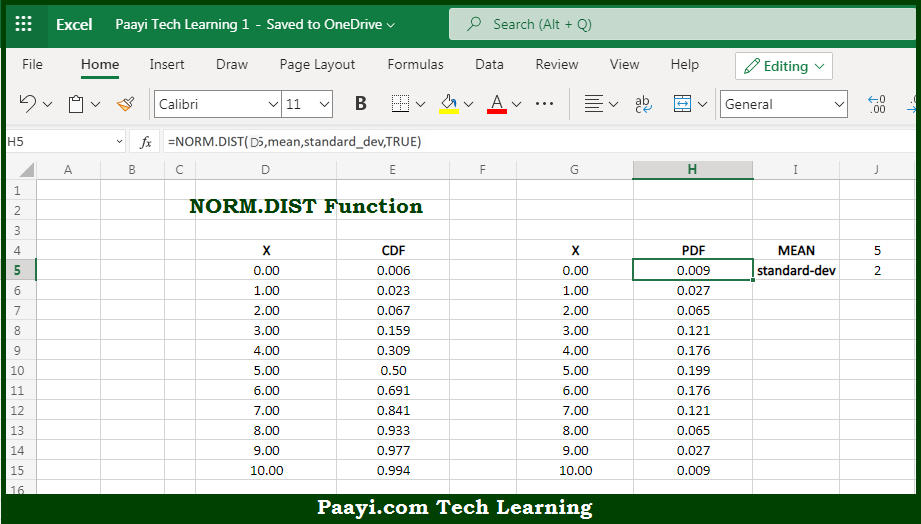# Learn How to Use Microsoft Excel NORM.DIST Function

Written by | 0 Comments | 648 Views

In this article, you will learn how to use the Microsoft Excel NORM.DIST function and its prime function in Microsoft Excel. You will also get to know the Microsoft Excel NORM.DIST function return value and syntax with the help of some examples.

Microsoft Excel NORM.DIST Function

The main purpose of the Microsoft Excel NORM.DIST function is to get the values and areas for the normal distribution. That implies, with the help of NORM.DIST function you can able to return the values for the normal probability density function (PDF) and the normal cumulative distribution function (CDF). The PDF returns values of points on the curve. The CDF returns the area under the curve to the left of a value. So, with the help of NORM.DIST function, you can able to get the values and areas for the normal distribution.

Return Value of NORM.DIST Function

The return value will be the output of the normal PDF and CDF.

Syntax of NORM.DIST Function

=NORM.DIST(x, mean, standard-dev, cumulative)

Where the arguments:

• x: This is the input value x.
• mean: This is the center of the distribution.
• standard-dev: This is the standard deviation of the distribution.
• cumulative: This is a boolean value that determines whether the probability density function or the cumulative distribution function is used.

## How to Use Microsoft Excel NORM.DIST Function?So we know that, Microsoft Excel NORM.DIST function you can able to get the values and areas for the normal distribution. That implies, with the help of NORM.DIST function you can able to return the values for the normal probability density function (PDF) and the normal cumulative distribution function (CDF). The PDF returns values of points on the curve. The CDF returns the area under the curve to the left of a value. So, with the help of NORM.DIST function, you can able to get the values and areas for the normal distribution.